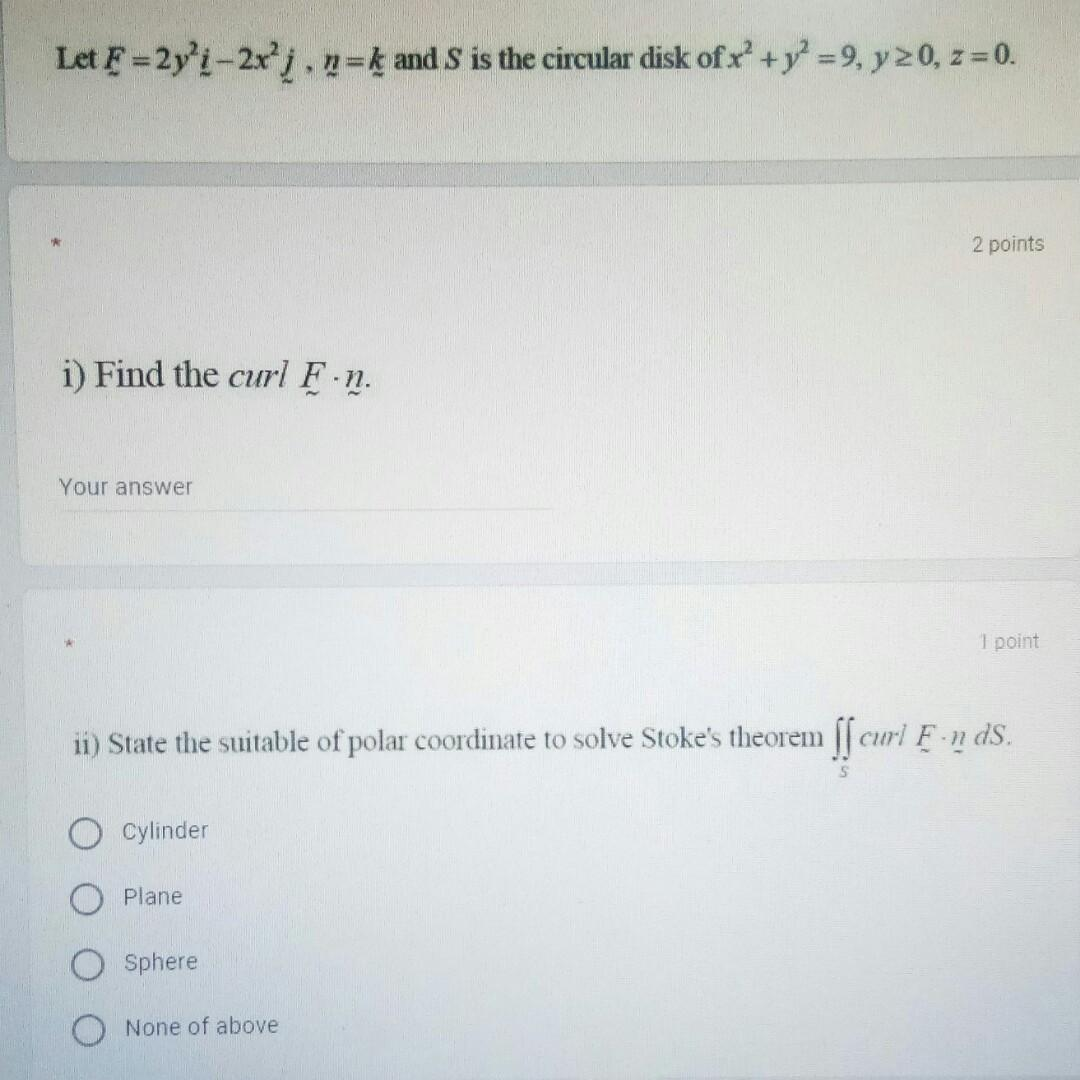### Create an Account

Already have account?

### Forgot Your Password ?

Home / Questions / Let F=2yʻi -2xj. n=k and S is the circular disk of r’ + y2 =9, y=0, z = 0. 2 points i) Fin...

# Let F=2yʻi -2xj. n=k and S is the circular disk of r’ + y2 =9, y=0, z = 0. 2 points i) Find the curl F.n. Your answer 1 point ii) State the suitable of polar coordinate to solve Stoke's theorem ſſ cur

Let F=2yʻi -2xj. n=k and S is the circular disk of r’ + y2 =9, y=0, z = 0. 2 points i) Find the curl F.n. Your answer 1 point ii) State the suitable of polar coordinate to solve Stoke's theorem ſſ curl F-n ds. Cylinder Plane Sphere None of aboveApr 14 2021 View more View Less

#### Answer (Solved)Subscribe To Get Solution